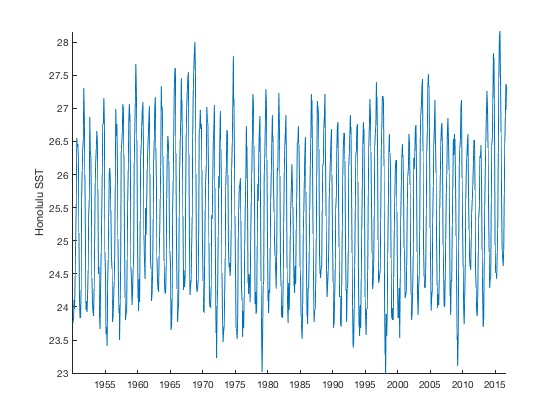The geomask returns true for locations within a specified geographic region.

Back to Climate Data Tools Contents

## Syntax

```mask = geomask(lat,lon,latv,lonv)

## Description

mask = geomask(lat,lon,latv,lonv) returns a mask the size of lat and lon that is true for all points within the bounds given by latv,lonv.

• scalar latv,lonv: If latv,lonv are scalar values, the output mask will be true for only the pixel closest to latv,lonv.
• two-element arrays: If latv,lonv are two-element arrays (e.g., [40 50],[110 120]) the output mask will be true for all lat,lon values within the geographic quadrangle bounded by latv,lonv.
• polygon defined by latv,lonv: If latv,lonv contain more than two elements, the output mask will be true for all elements of lat,lon within the polygon defined by latv,lonv.
• polygons in cell format: If latv,lonv are cell arrays (as is common for multiple areas in a shapefile), the output mask is true for all elements within any of the polygons in latv,lonv.

mask = geomask(lat,lon,latv,lonv,'inclusive') includes lat,lon points that are on the boundary or boundaries defined by latv,lonv.

[mask,coords] = geomask(...) If latv,lonv are scalar, the optional coords output is a structure containing the coordinates of the pixel in the mask. The coords structure includes coords.row and coords.col which are the row and column of of the lat,lon grid, and coords.lat and coords.lon, which are the geographic location of the output grid cell.

Sometimes you're interested in values that are withing a geographic quadrangle. One such quadrangle is the Nino 3.4 box is defined as (5°S to 5°N, 170°W to 120°W). Here's some sample sea surface temperature data

```% Load some sample data:

% Plot the sample data:
imagescn(lon,lat,mean(sst,3))
axis xy image
cmocean thermal
xlabel 'longitude'
ylabel 'latitude'

% Define the Nino 3.4 box:
latv = [-5 -5 5 5 -5];
lonv = [-170 -120 -120 -170 -170];

% Plot the Nino 3.4 box:
hold on
plot(lonv,latv,'k-','linewidth',2)
```For a simple geographic quadrangle the easiest way to get a mask is to define the limits of the bounding box (similar to ingeoquad if you have Matlab's Mapping Toolbox).

Start by converting the lat,lon arrays into a grid, then get the mask by specifing the limits of the region interest:

```% Convert lat,lon into a grid:
[Lon,Lat] = meshgrid(lon,lat);

figure
xlabel 'longitude'
ylabel 'latitude'
title 'yellow indicates *true* grid cells (the Nino 3.4 box)'
```## Example 2: Nearest grid cell

Sometimes you need a time series near a single point of interest. For example, you might want to plot the SST time series near Honolulu (21.3 N, 157.8 W). Let's find the grid cell closest to Honolulu:

```[honolulu,coords] = geomask(Lat,Lon,21.3,-157.8);

figure
imagescn(lon,lat,honolulu)
xlabel 'longitude'
ylabel 'latitude'
hold on
borders % plots national boundaries for context
```A single-pixel mask may be of limited use, but for such masks, geomask also offers the row, column, and geographic locations of the nearest pixel, see:

```coords
```
```coords =
struct with fields:

row: 20
col: 12
lat: 21.5000
lon: -157.5000
```

As proof, below we plot the mean SST map with a red star over our desired Honolulu location and a blue square over the grid cell closest to Honolulu:

```% Plot the mean SST for context:
figure
imagescn(lon,lat,mean(sst,3))
axis xy
cmocean thermal
xlabel 'longitude'
ylabel 'latitude'

% Plot Honolulu as a red star and its nearest grid cell as a blue square:
hold on
plot(-157.8,21.3,'rp')
plot(coords.lon,coords.lat,'bs')
text(-157.8,21.3,' Honolulu','color','r','vert','top')
text(coords.lon,coords.lat,'  nearest grid cell','color','b','vert','bottom')
```And with the row and column information in the coords output, it's easy to get a time series for the Honolulu grid cell. Note that sst is a 3D matrix, so we have to use the squeeze command to remove singleton dimensions. For clarity, I

```sst_honolulu = sst(coords.row,coords.col,:);

% remove singleton dimensions:
sst_honolulu = squeeze(sst_honolulu);

figure
plot(t,sst_honolulu)
axis tight
box off
ylabel('Honolulu SST')
datetick('x','keeplimits')
```## Example 3: Arbitrarily-shaped polygon(s)

If you want to make a mask of an arbitrary polygon or multiple polygons, you can use the standard Matlab function inpolygon, or you can let geomask do it for you. With geomask, latv and lonv can be numeric arrays just like you would use with inpolygon, or latv and lonv can be cell arrays with polygons in each cell.

In this example, we have a global grid and we want to know which grid cells are within the borders of Latin American countries. CDT comes with sample borderdata, and by going through the country names, you can manually pick out which countries correspond to Latin America. Below I've picked 20 Latin American countries which we'll use in to make the mask.

This example may take a couple of seconds to compute because the national outlines in borderdata are somewhat high resolution and it takes time for geomask to compare the grid cells to the outline of 41,500 border data vertices.

```% For this sample 1 degree resolution grid:
[Lat,Lon] = geogrid;

% Load some national border data:

% Use only Latin American countries:
latv = B.lat([8 17 21 33 38 39 41 48 49 55 75 78 79 120 159 161 162 165 211 214]);
lonv = B.lon([8 17 21 33 38 39 41 48 49 55 75 78 79 120 159 161 162 165 211 214]);

% Find grid cells corresponding to Latin American countries: